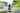philschmid blog

# K-Fold as Cross-Validation with a BERT Text-Classification Example

#Python #Bert

### Philipp Schmid

, April 07, 2020 · 4 min readPhoto by Martin Sanchez on Unsplash

K-fold is a cross-validation method used to estimate the skill of a machine learning model on unseen data. It is commonly used to validate a model, because it is easy to understand, to implement and results are having a higher informative value than regular Validation Methods.

Cross-validation is a resampling procedure used to validate machine learning models on a limited data set. The procedure has a single parameter called K that refers to the number of groups that a given data sample is to be split into, that’s the reason why it´s called K-fold.

The choice of K is usually 5 or 10, but there is no formal rule. As K is getting larger, the resampling subsets are getting smaller. The number of K also defines how often your Machine Learning Model is trained. Most of the time we split our data into train/validation sets in 80%-20%, 90%-10% or 70%-30% and train our model once. In cross-validation, we split our model K times and then train. Be aware that this will result in longer training processes.

## K-Fold steps:

1. Shuffle the dataset.
2. Split the dataset into K groups.
3. For each unique group g:
1. Take g as a test dataset.
2. Take the remaining groups as a training data set.
3. Fit a model on the training set and evaluate it on the test set.
4. Retain the evaluation score and discard the model.
4. Summarize the skill of the model using the sample of model evaluation scores.The results of a K-fold cross-validation run are often summarized with the mean of the model scores.

## Scitkit-Learn Example

The example is a simple implementation with scikit-learn and a scalar numpy array .

1 import numpy as np2     from sklearn.model_selection import KFold3     # data sample4     data = np.array([0.1, 0.2, 0.3, 0.4, 0.5, 0.6])5     # prepare cross validation6     kfold = KFold(n_splits=3, shuffle=True, random_state=1)7     # enumerate splits8     for train, test in kfold.split(data):9         print('train: %s, test: %s' % (data[train], data[test]))10 11     #>>> Result12     #train: [0.1 0.4 0.5 0.6], test: [0.2 0.3]13     #train: [0.2 0.3 0.4 0.6], test: [0.1 0.5]14     #train: [0.1 0.2 0.3 0.5], test: [0.4 0.6]

## Simpletransformers Example (BERT Text-Classification)

The example is an implementation for a BERT Text-Classification with simpletransformers library and Scikit-Learn.

1 from simpletransformers.classification import ClassificationModel2     from sklearn.model_selection import KFold3     from sklearn.metrics import accuracy_score4     import pandas as pd5 6 7     # Dataset8     dataset = [["Example sentence belonging to class 1", 1],9                          ["Example sentence belonging to class 0", 0],10                          ["Example eval sentence belonging to class 1", 1],11                          ["Example eval sentence belonging to class 0", 0]]12     train_data = pd.DataFrame(dataset)13 14     # prepare cross validation15     n=516     kf = KFold(n_splits=n, random_state=seed, shuffle=True)17 18     results = []19 20     for train_index, val_index in kf.split(train_data):21             # splitting Dataframe (dataset not included)22         train_df = train_data.iloc[train_index]23         val_df = train_data.iloc[val_index]24         # Defining Model25         model = ClassificationModel('bert', 'bert-base-uncased')26             # train the model27         model.train_model(train_df)28             # validate the model29         result, model_outputs, wrong_predictions = model.eval_model(val_df, acc=accuracy_score)30         print(result['acc'])31             # append model score32         results.append(result['acc'])33 34 35     print("results",results)36     print(f"Mean-Precision: {sum(results) / len(results)}")37 38     #>>> Result39     # 0.853578463558765540     # 0.850952068286277141     # 0.85554826001313242     # 0.827201051248357443     # 0.821287779237844944     #results [0.8535784635587655,0.8509520682862771,0.855548260013132,45     #                    0.8272010512483574,0.8212877792378449]46     # Mean-Precision: 0.8407520682862771

## Benefits of K-Fold Cross-Validation

Using all data: By using K-fold cross-validation we are using the complete dataset, which is helpful if we have a small dataset because you split and train your model K times to see its performance instead of wasting X% for your validation dataset.

Getting more metrics: Most of the time you have one result of metrics, but with K-Fold you´ll be able to get K results of the metric and can have a deeper look into your model’s performance.

Achieving higher precision: By validating your model against multiple “validation-sets” we get a higher level of reliability. Let’s imagine the following example: We have 3 speakers and 1500 recordings (500 for each speaker). If we do a simple train/validation split the result could be very different depending on the split.

### Join my email list and get notified about new content

Be the first to receive my latest content with the ability to opt-out at anytime. I promise to not spam your inbox or share your email with any third parties.